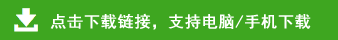# 苏教版一年级数学上册期中试题

一、直接写出得数。（20分）

4-4=       2+3=        5-4=        4-0=       3+5=
4+2=       7-3=        0+7=        6+1=      6-3=
4-2=       2+3=        3-2=        5+1=       7-4=
6-6=       2+2=        4-3=        2-2=       7-1-4=
二、填一填。（32分）
1、看图写数。
(     )颗
2、
（1）一共有（    ）个图形，其中有（    ）个    。
（2）的左边有（    ）个图形，右边有（    ）个图形。
（3）从右数第（    ）个和第（    ）个是     。
3、在□里填上合适的数
（1）上面的数中最大的是（    ），与4相邻的数是（    ）和（    ）。
（2）比7大，比10小的数有（     ）个。
4、在〇里填“>”、“<”或“=”。
9〇6          6-6〇5        5+3〇3+5
5、在（    ）里填上合适的数。
2+（  ）=5      7-（  ）=0      (   )+0=4
6、找规律填数。
（1）
（2）
7、  ☆☆☆☆☆☆☆
◇◇◇◇
△△△△△△
8、如果一次只能端两盘，那么一次最多能端走（     ）个梨，最少能端走（     ）个梨。